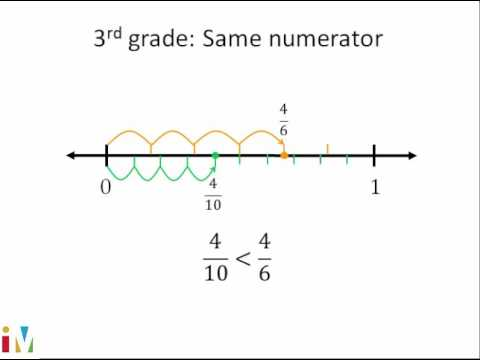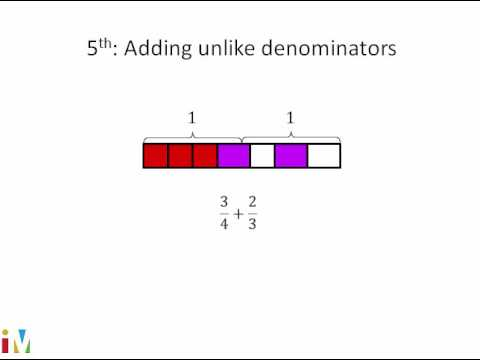Video

# Multiplying 2 fractions: fraction model (Full video)

Khan Academy

## Supporting grades: 5

Description: Here's a great way to visualize fraction multiplication problems. Once you understand this, doing them on your own will be a lot easier! Let's think a little bit about what it means to multiply fractions. Say I want to multiply 1/2 times 1/4. So let me divide it into fourths, so I'll divided into 4 equal sections.

You must log inorsign upif you want to:*

*Teacher Advisor is 100% free.

### Other videos you might be interested in### Comparing Fractions

#### Illustrative Mathematics